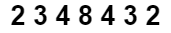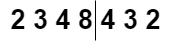# Check if a number is a palindrome or not without using any extra space.

## Introduction

This blog will discuss the step-by-step process to check if a number is a palindrome or not without using any extra space.

So, before jumping into the problem, let’s first understand a palindrome.

What is a Palindrome?

A palindrome is a number, word, phrase, or sequence of characters that reads the same forwards as backwards, such as racecar, malayalam, 432234 etc.

If we reverse a palindrome, it will be the same as the original palindrome.

### Problem statement

We are given a number. Our task is to identify whether the given number is a palindrome or not without using any extra space.

### Sample examples

Input1: n = 2345432

Output1: YES

Explanation:

Original:  2345432

After reversing: 2345432

The given number is the same as the reversed number; it is a palindrome.

Input2: 33548

Output2:  NO

Explanation:

Original: 33543

After reversing: 84533

The given number is not the same as the reversed number; it is not a palindrome number.

## Approach

The idea is simple, we divide the number into two halves and reverse the second half. If both are the same, it is a palindrome number as it reads the same forwards as backwards; otherwise, it is not a palindrome number.

To divide the number into two halves, we count the number of digits in the given number.

There is a case when the number of digits in the given number is odd. In this case, we will remove the last number from the first half number.

Steps:

• If the given number is negative, print NO.
• If the given number is less than 10, print YES as the single-digit number is a palindrome.
• Create a digits variable and store the number of digits in it.
• Create a half variable to store the second half number, initialise it with zero.
• Run a for loop (digits/2) times and do half = half * 10 + n%10 and n = n/10; it stores the second half number in the reversed form in half variable.
• The second half number is currently stored in the half variable, and the first half is stored in n.
• If the number of digits is odd, remove the last number from the first half.
• Finally, check whether the first half is equal to the second half or not. If both are equal, print YES else, print NO.

Let’s understand the above approach with an example:

Given number:Number of digits = 7

After dividing the number into two halves :The first half number = 2348

The second half number = 234

Now, the number of digits is odd; remove the last number from the first half.

The first half after removing the last number = 234

The first half number is the same as the second half number. So, it is a palindrome number.

### Implementation in C++

``````#include <bits/stdc++.h>
using namespace std;

void palindrome_number(int n)
{
if (n < 0)
cout << "NO";
if (n < 10)
cout << "YES";

int digits = (log(n) / log(10)) + 1;

int half = 0;

for (int i = 0; i < digits / 2; i++) {
half = half * 10 + n % 10;
n = n / 10;
}

if (digits % 2 != 0 )
half = half * 10 + n % 10;

if (half == n)
cout << "YES";
else
cout << "NO";
}

int main()
{
int n;

cin >> n;

palindrome_number(n);

return 0;
}``````

Input

``8175718``

Output

``YES``

#### Complexity Analysis

Time complexity: O(digits) as we used a for loop of size digits/2.

Space complexity: O(1) as we have used constant extra space.

Q1. How can you tell if a number is a palindrome?

Ans:

• Declare two variables, one for the given number and the other for the reversal.
• Continue running the do-while loop until the number of digits in the reversed number equals the number of digits in the given number.
• Check if the reversed number is the same as the given one.

Q2. How do you determine whether a rearranged number is a palindrome?

Ans: A palindrome is formed when at least one character appears an odd number of times and all other characters appear an even number of times. Running two loops, the outer loop picking all characters one by one and the inner loop counting the number of occurrences of the picked character, is a simple solution.

## Key Takeaways

This article discussed palindrome and the approach to check if a number is a palindrome or not without using extra space with examples to better understand its C++ code.

If you are a beginner, interested in coding and want to learn DSA, you can look for our guided path for DSA, which is free!